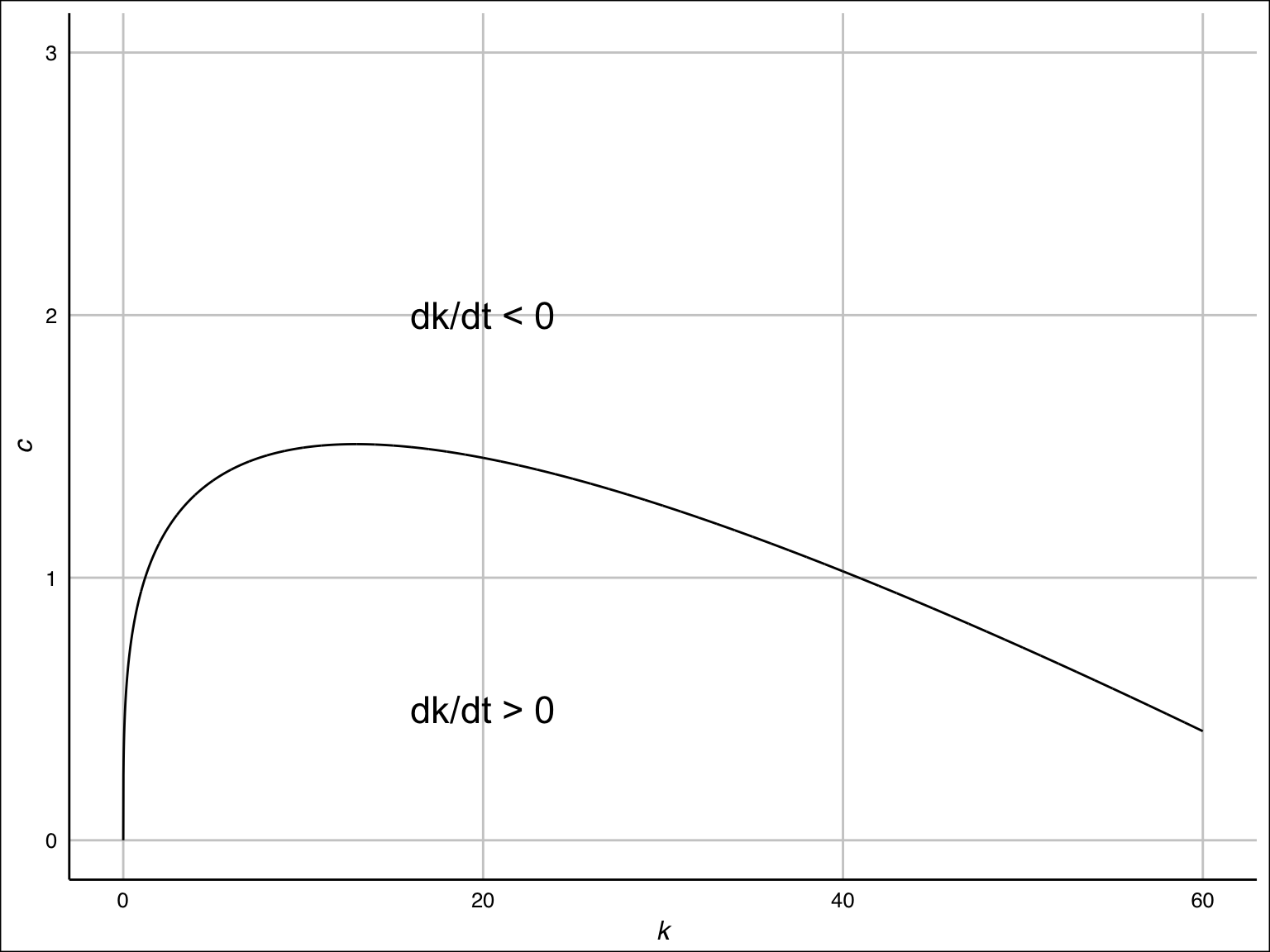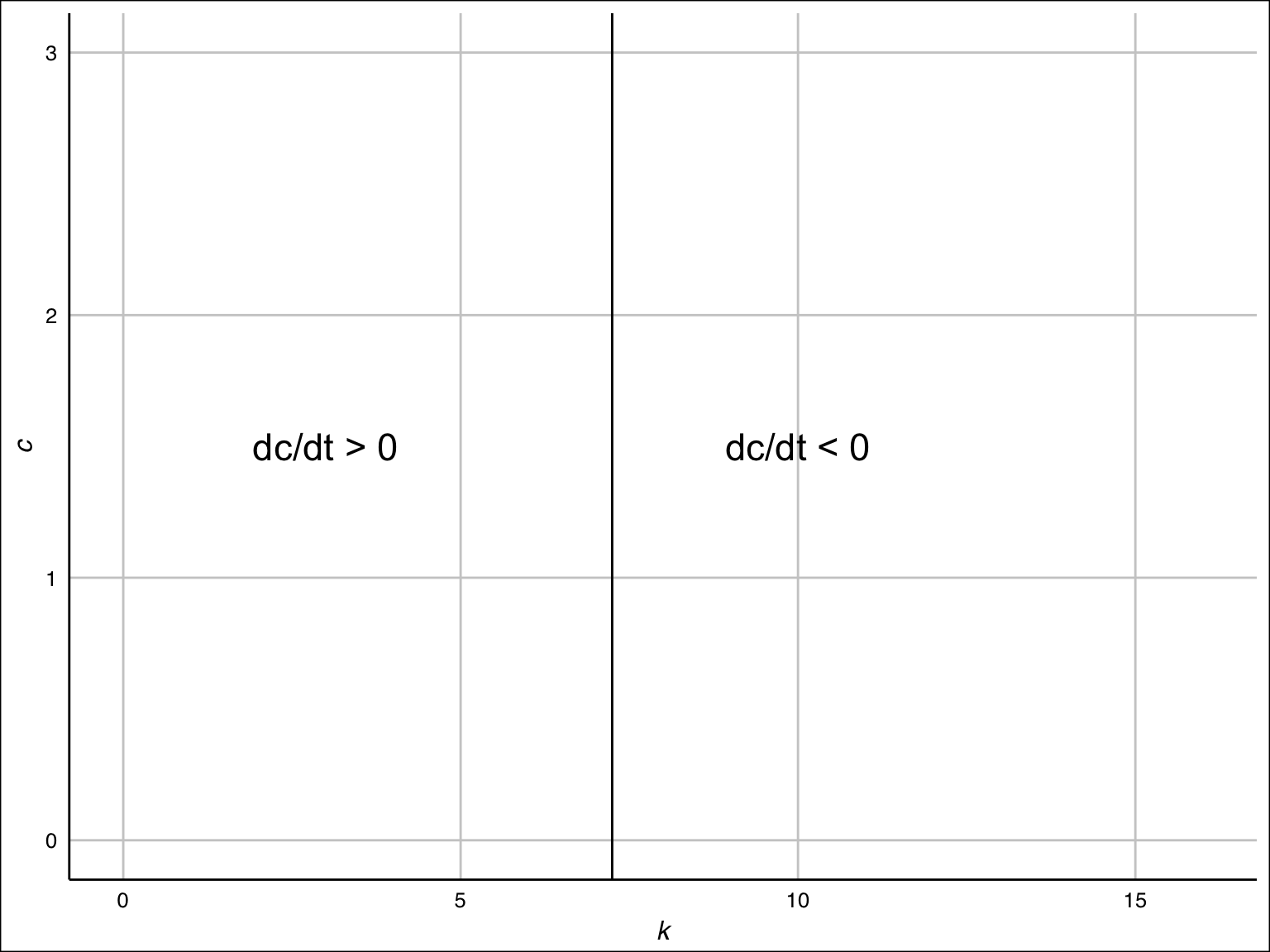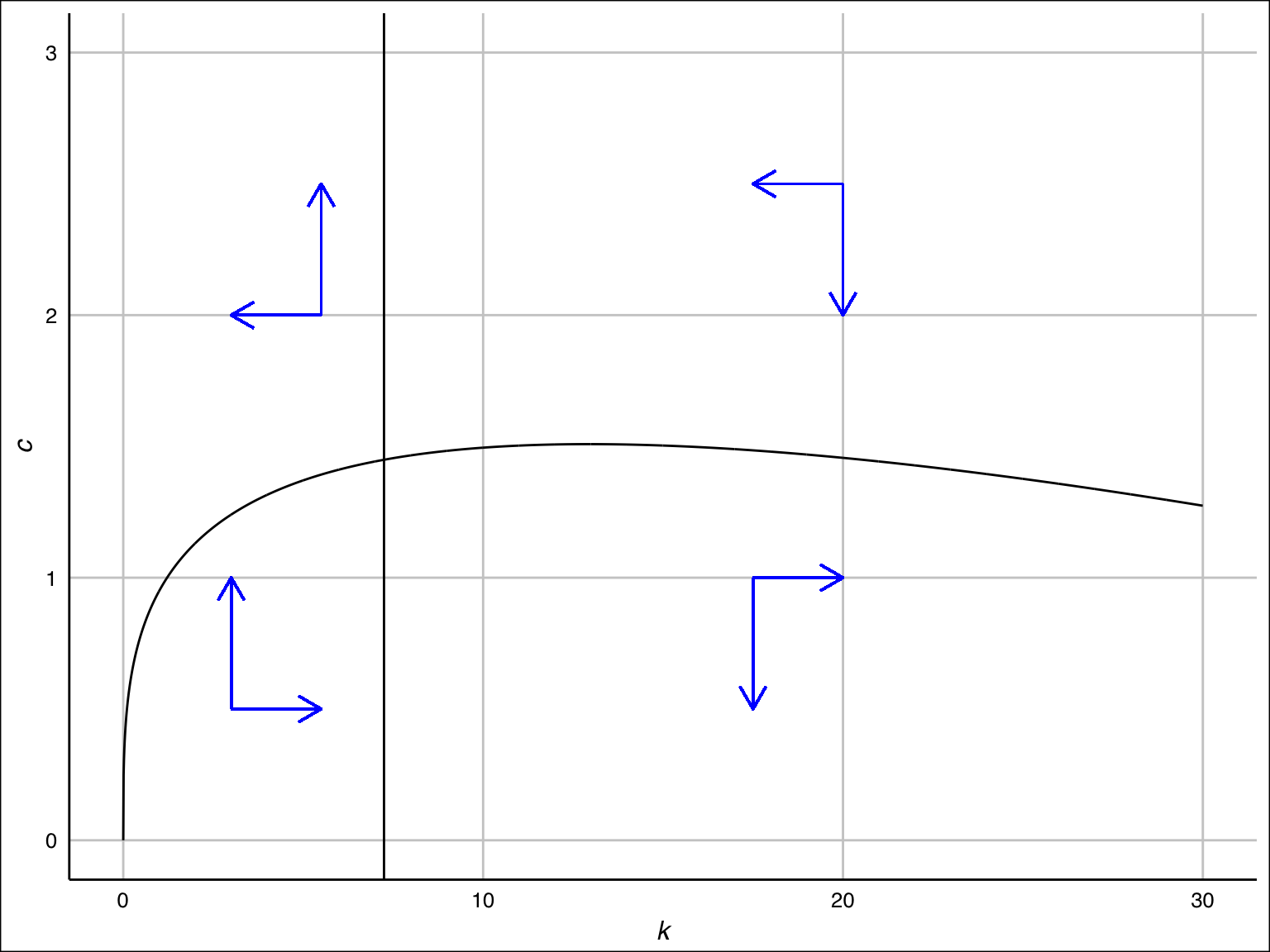January 11, 2017

# Optimal Growth

\begin{aligned} & \max \int_0^\infty e^{-\rho t} u(c(t)) dt \\ &\text{subject to } \\ &\qquad c(t) = f(k(t)) - \delta k(t) - \dot k(t) \ge 0, \\ &\qquad k(t) \ge 0, \\ &\qquad k(0): \text{given}. \end{aligned}

# Euler Equation

As you will see later, the full dynamics of $$c(\cdot)$$ and $$k(\cdot)$$ is governed by

\begin{aligned} \dot k (t) &= f(k(t)) - \delta k(t) - c(t) \\ \dot c (t) &= \frac{u'(c(t))[f'(k(t)) - \delta - \rho]}{- u''(c(t))} = \frac{u'(c(t))(r(t) - \rho)}{- u''(c(t))}, \end{aligned}

where $$r(t) = f'(k(t)) - \delta$$ is net rate of return on capital.

When the rental rate (instantaneous reward of owning capital) is greater than the discount rate (instantaneous cost of not consuming), the representative agent decides to spend this profits to increase consumption somewhat.

# Transversality condition

It is known that, under a mild set of conditions (See Kamihigashi 2001, Econometrica), transversality condition (not die before the present value of capital is zero)

$\lim_{t\to\infty}-e^{-\rho t}u'(c(t))k(t)=0$

is necessary for optimality; i.e., among infinitely many paths that satisfy the above mentioned system of differential equations, those that satisfy the transversality condition are optimal.

In our case, we can specify only one solution.

$$e^{-\rho t}u'(c(t)) k(t)$$ is the present value of period-$$t$$ capital. The present value of positive amount of capital in the infinite future should be zero. If it’s positive, $$u'(c(t))k(t)$$ grows indefinitely and the representative agent cannot maximize his utility.

A candidate of the solution is the path that converges to a constant; i.e.,

$(k(t), c(t)) \to (k^*, c^*)$ Since $$e^{-\rho t} \to 0$$ and $$u'(c(t))k(t) \to u'(c^*) k^*$$, we have the transversality condition.

# Conditions for the steady state

\begin{aligned} c &= f(k) - \delta k \\ k &= (f')^{-1} (\delta + \rho). \end{aligned}

Exercise: Show that the steady state $$(k^*, c^*)$$ satisfies the above system of equations. [Hint: What was the definition of steady state?]

# $$\dot k \gtreqqless 0$$

\begin{aligned} \dot k > 0 &\Leftrightarrow f(k) - \delta k - c > 0 \\ &\Leftrightarrow c < f(k) - \delta k \end{aligned}

\begin{aligned} \dot k < 0 &\Leftrightarrow f(k) - \delta k - c < 0 \\ &\Leftrightarrow c > f(k) - \delta k \end{aligned}

# $$\dot k \gtreqqless 0$$# $$\dot c \gtreqqless 0$$

\begin{aligned} \dot c > 0 &\Leftrightarrow f'(k) - \delta - \rho > 0\\ &\Leftrightarrow k < (f')^{-1}(\delta + \rho) \end{aligned}

\begin{aligned} \dot c < 0 &\Leftrightarrow f'(k) - \delta - \rho > 0\\ &\Leftrightarrow k > (f')^{-1}(\delta + \rho) \end{aligned}

# $$\dot c \gtreqqless 0$$# Phase Diagram# Hamiltonian Method: The target problem

The most frequently used method for continuous-time problems is the Hamiltonian method. Consider the following problem (somewhat more abstract than the optimal growth)

\begin{aligned} &\max\int_{0}^{\infty}F(k,c,t)dt \\ &\text{subject to} \\ &\qquad\dot{k}=G(k,c,t), \\ &\qquad k(0):\ \text{given,} \end{aligned}

# Hamilotonian Method: Hamiltonian

The Hamiltonian (or more precisely, present-value Hamiltonian) is the following function.

$\mathcal{H}(k, c, \lambda, t) =F(k,c,t)+\lambda G(k,c,t),$

where $$\lambda = \lambda(t)$$ is a multiplier.

# Hamiltonian Method: Canonical Equations

The solution of the problem must satisfy (necessary condition), $$\frac{\partial\mathcal{H}}{\partial c} =0$$,

\begin{aligned} \frac{\partial\mathcal{H}}{\partial c} &= 0 \\ \dot{\lambda} &=-\frac{\partial\mathcal{H}}{\partial k},\\ \dot{k} &= \frac{\partial\mathcal{H}}{\partial\lambda}. \end{aligned}

We do not see the proof here. See Acemoglu (2009) if you are interested.

These conditions plus the transversality conditions give you the optimal path.

# Hamiltonian Method: Interpretations (or how to memorize)

\begin{aligned} \frac{\partial\mathcal{H}}{\partial c} &= 0, \\ \dot{\lambda} &=-\frac{\partial\mathcal{H}}{\partial k},\\ \dot{k} &= \frac{\partial\mathcal{H}}{\partial\lambda}. \end{aligned}

1. Roughly speaking, the Hamilotonian represents the Total Utility remaining at $$t$$.
2. The first equation states that the control is chosend to maximize the total utility.
3. $$\lambda(t)$$ represents the present price of capital at $$t$$ and the second equation states that the instantaneous increment of price is characterized by the marginal value (marginal product measure in the unit of present utlity price)
4. The third equation is exactly the resource constraint.

# Exercise

Dynamic optimization problems: $\begin{array}{l} &\max\int_{0}^{\infty}F(k,c,t)dt \\ &\text{subject to} \\ &\qquad\dot{k}=G(k,c,t), \\ &\qquad k(0):\ \text{given,} \end{array} \quad\leftrightarrow\quad \begin{array}{l} & \max \int_0^\infty e^{-\rho t} u(c) dt \\ &\text{subject to } \\ &\qquad c = f(k) - \delta k - \dot k, \\ &\qquad k(0): \text{given}. \end{array}$ Define the Hamiltonian $\mathcal{H} =F(k,c,t)+\lambda G(k,c,t), \quad\longleftrightarrow\quad ???$

Obtain the first-order conditions: $\begin{array}{rl} \frac{\partial\mathcal{H}}{\partial c} &= 0 \\ \dot{\lambda} &=-\frac{\partial\mathcal{H}}{\partial k},\\ \dot{k} &= \frac{\partial\mathcal{H}}{\partial\lambda}. \end{array} \quad\longleftrightarrow\quad \begin{array}{rl} \dot k (t) &= f(k) - \delta k - c \\ \dot c (t) &= \frac{u'(c)[f'(k) - \delta - \rho]}{- u''(c)}, \end{array}$ You have 15 minutes and prove the first-order dynamical system of the optimal growth model.